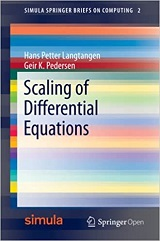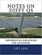Processing ......FreeComputerBooks.com Links to Free Computer, Mathematics, Technical Books all over the World

Scaling of Differential Equations
Want to know the Homepage of a particular airport? Click here to find out.
• Title: Scaling of Differential Equations
• Author(s) Hans Petter Langtangen, Geir K. Pedersen
• Publisher: Springer; 1st ed. (June 24, 2016); eBook (Creative Commons Licensed)
• Paperback: 151 pages
• eBook: PDF
• Language: English
• ISBN-10: 3319327259
• ISBN-13: 978-3319327259Book Description

Scaling is a classical topic in applied mathematics, but here strongly connected to numerical simulations. The book contains a wide range of examples, of differing complexity, from many different scientific fields.

The book serves both as a reference for various scaled models with corresponding dimensionless numbers, and as a resource for learning the art of scaling.

• N/A
Reviews, Ratings, and Recommendations: Related Book Categories: Read and Download Links:Similar Books:
•Notes on Diffy Qs: Differential Equations for Engineers (Jiri Lebl)

An introductory course on differential equations aimed at engineers. The book covers first order ODEs, higher order linear ODEs, systems of ODEs, Fourier series and PDEs, eigenvalue problems, the Laplace transform, and power series methods.

•Finite Difference Computing with PDEs: A Software Approach

This easy-to-read book introduces the basics of solving partial differential equations by means of finite difference methods. Unlike many of the traditional academic works on the topic, this book was written for practitioners.

•A Friendly Introduction to Differential Equations (M. Kaabar)

Very useful for college students who studied Calculus II, and other students who want to review some concepts of differential equations before studying courses such as partial differential equations, applied mathematics, and electric circuits II.

•Solving PDEs in Python: The FEniCS Tutorial I (H. Langtangen)

This book offers a concise and gentle introduction to finite element programming in Python based on the popular FEniCS software library. Using a series of examples, it guides readers through the essential steps to quickly solving a PDE in FEniCS.

Book Categories
 :All CategoriesRecent BooksMiscellaneous BooksComputer LanguagesComputer ScienceData Science/DatabasesElectrical EngineeringJava and Java EE (J2EE)Linux and UnixMathematicsMicrosoft and .NETMobile ComputingNetworking and CommunicationsSoftware EngineeringSpecial TopicsWeb Programming
Other Categories